# How to make a Mandelbulb

Quickly visualise a 3D fractal in Houdini using an SDF.

A Mandelbulb is a three-dimensional fractal that is becoming increasingly popular in 3D art and VFX. In this article, I’ll walk through how to quickly make a Mandelbulb in Houdini, using an SDF (signed distance field volume).

In each voxel, an SDF stores the distance to the nearest point on the surface. Houdini can interpret these distances, and visualise the derived surface as 3D geometry. This is very convenient since most fractal formulas take the form of a ‘distance estimation function’ – given a point in space, the function returns the distance to the nearest point on the fractal implicit surface. This means that you can put the results of the fractal function directly in each voxel and it will just work!

### 01. Create an empty volume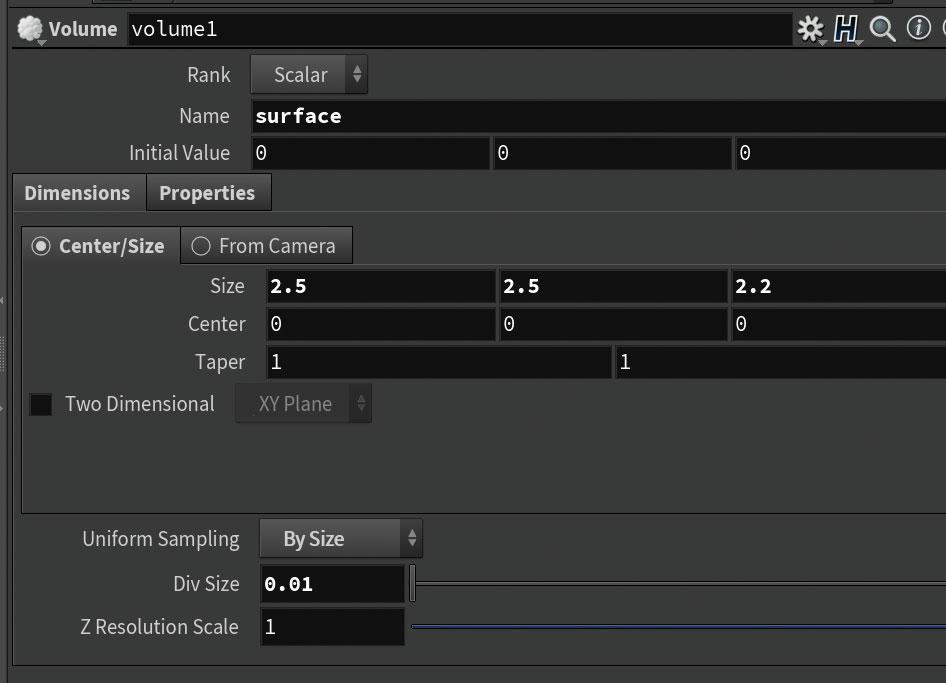Click the icon in the top right to enlarge the image

First, create an empty volume (Volume SOP) to fill with distance values – give it the name 'surface'. A good fractal to test with is the Mandelbulb, and by default it's around 2.5 units wide, so set the volume's size to 2.5, 2.5, 2.5. You can change the resolution of the volume to trade off speed against quality; a good starting point could be setting the Uniform Sampling to By Size, with a Division Size of 0.01. The default mode of visualising a volume in Houdini is a fog volume, but you can change that to display as a hard limit surface, by changing the Display Mode to Isosurface (in the Properties tab).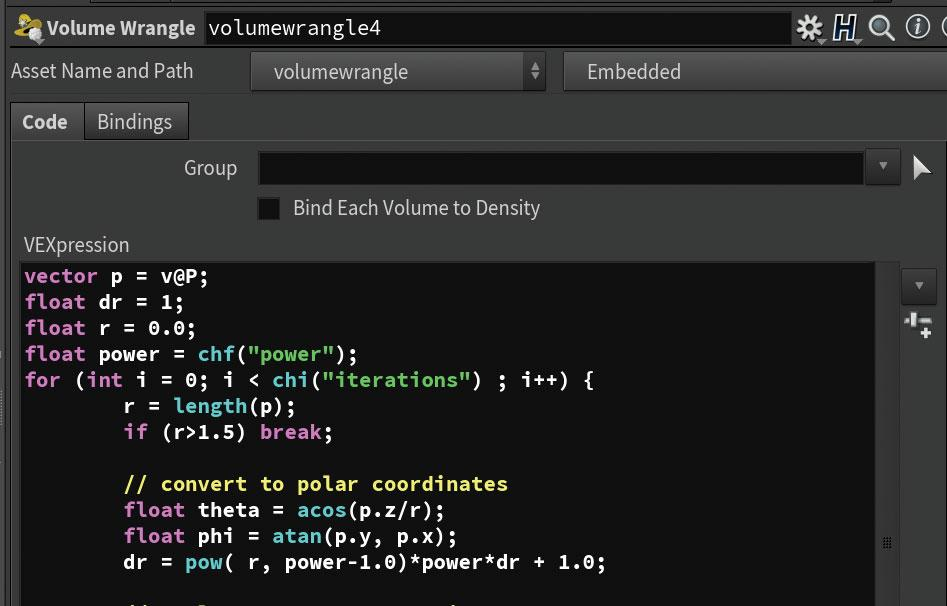Click the icon in the top right to enlarge the image

Then it’s a matter of filling the voxels with distance values. Use this VEX code in a Volume Wrangle SOP to run the Mandelbulb formula per-voxel:

vector p = v@P;float dr = 1;float r = 0.0;float power = chf(“power”);for (int i = 0; i < chi(“iterations”) ; i++) { r = length(p); if (r>1.5) break; // convert to polar coordinates float theta = acos(p.z/r); float phi = atan(p.y, p.x); dr = pow( r, power-1.0)*power*dr + 1.0; // scale and rotate the point float zr = pow(r, power); theta *= power; phi *= power; // convert back to cartesian coordinates p = zr*set(sin(theta)*cos(phi), sin(phi)*sin(theta), cos(theta)); p += v@P; }f@surface = 0.5*log(r)*r/dr;

### 03. Introduce parameter references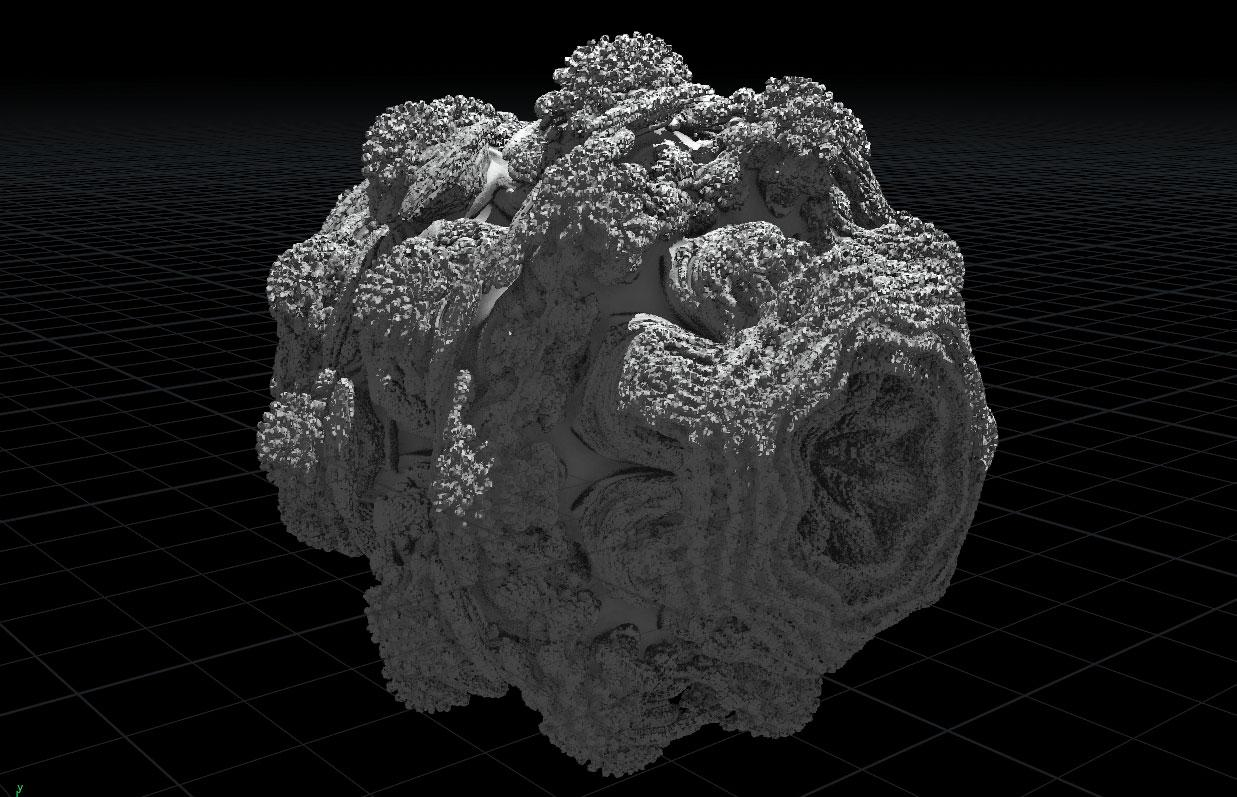Click the icon in the top right to enlarge the image

Houdini lets you add your own custom spare parameters to an individual node’s user interface. Our VEX code contains parameter references (the chf() functions), to let us control aspects of the formula with parameters in the UI. Those parameters won't exist by default, but you can create them by pressing the Create Spare Parameters button in the right of the code window.

In this case, the Iterations parameter will control the level of detail for the Mandelbulb – start off with 5 or 6. The Power will control the shape of the Mandelbulb – the typical shape emerges at around Power 8.0.

### 04. Convert to polygons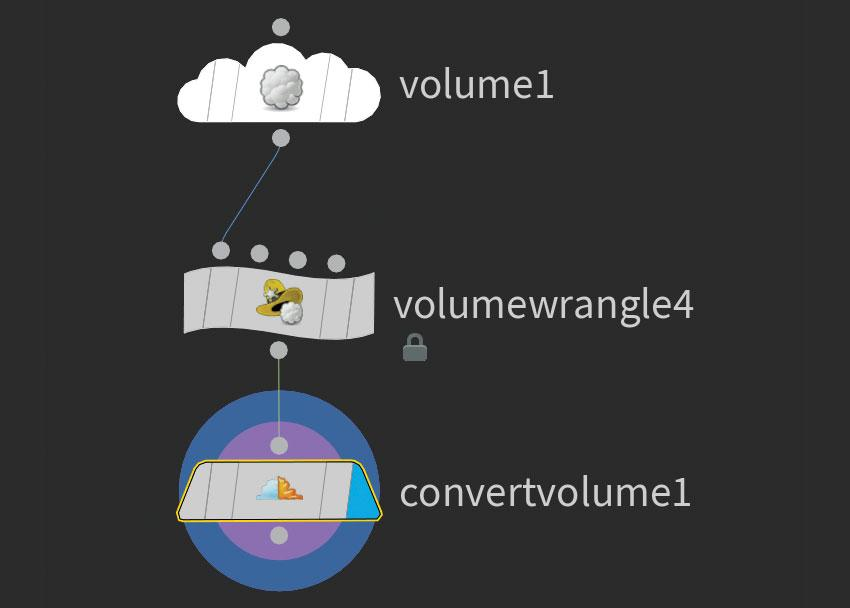Click the icon in the top right to enlarge the image

To convert the surface to polygons, follow the Volume Wrangle with a Convert Volume SOP. Use Invert mode, because it's interpreted as a signed distance field, and you'll get the detailed fractal shape meshed in polygon form. Effectively, the Invert mode reverses the normals of the surface and the winding direction of the polygons.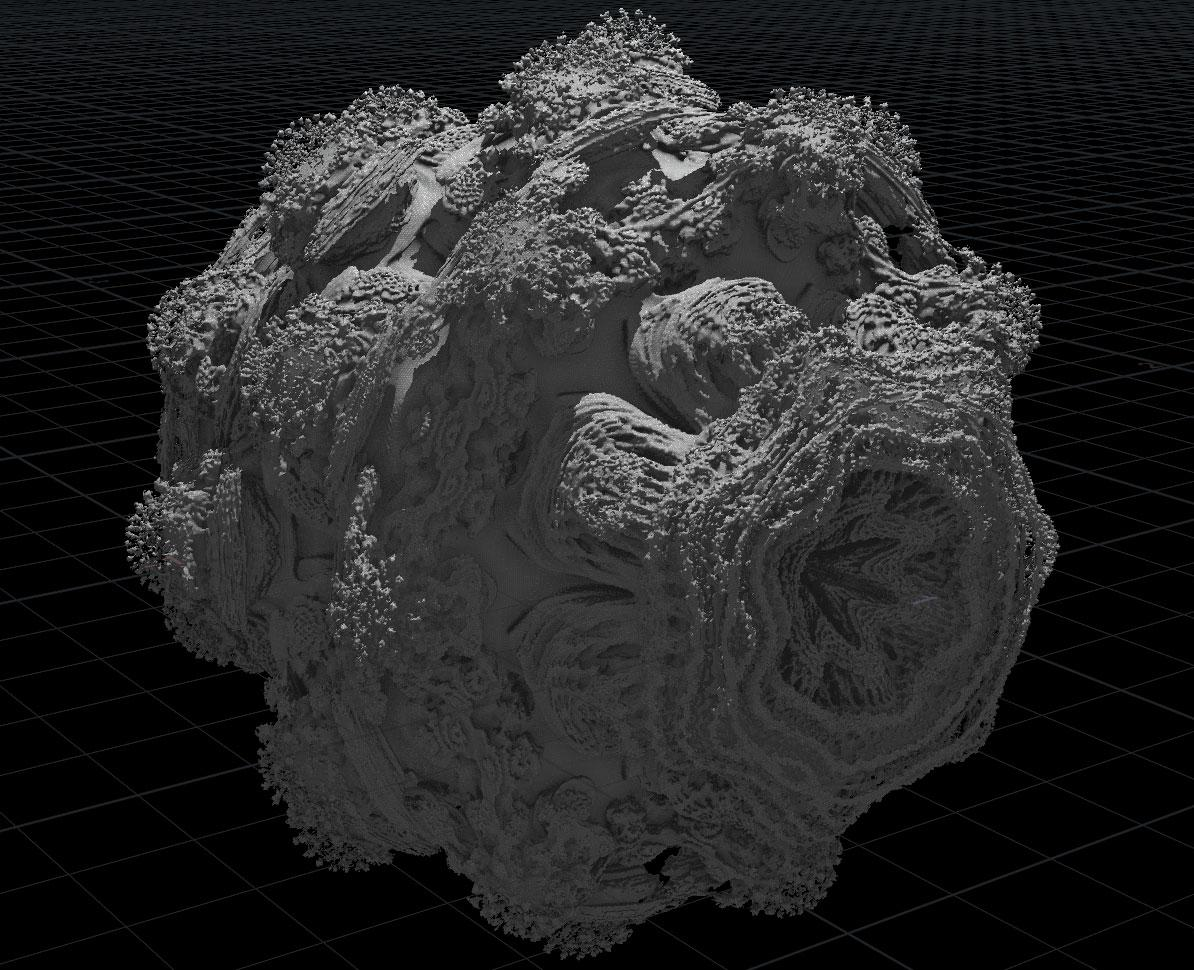Click the icon in the top right to enlarge the image

You can then render it the same as you would any other mesh, but because it's so detailed you won't be able to UV unwrap it easily – consider using procedural techniques to surface it, for example, shading based on its curvature.

This article was originally published in 3D World, the world's best-selling magazine for CG artists. Buy issue 237 or subscribe.